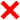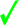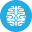# PHP Language | 10 Minute‐Test 8

Instruction

• Total number of questions : 10.
• Time alloted : 10 minutes.
• Each question carry 1 mark.
• No Negative marks
• DO NOT refresh the page.
• All the best :-).

1.

What will be the output of the following php code?

<?php

\$num  = 1;

\$num1 = 2;

print \$num . "+". \$num1;

?>

A.
 3B.
 1+2C.
 1.+.2D.
 ErrorCorrect Answer: Option B

Explanation:

.(dot) is used to combine two parts of the statement. Example ( \$num . “Hello World” ) will output 1Hello World.

2.

Which statement will output \$x on the screen?

A.
 echo “\\$x”;B.
 echo “\$\$x”;C.
 echo “/\$x”;D.
 echo “\$x;”;Correct Answer: Option A

Explanation:

A backslash is used so that the dollar sign is treated as a normal string character rather than prompt PHP to treat \$x as a variable. The backslash used in this manner is known as escape character.

3.

If \$a = 12 what will be returned when (\$a == 12) ? 5 : 1 is executed?

A.
 12B.
 1C.
 ErrorD.
 5Correct Answer: Option D

Explanation:

?: is known as ternary operator. If condition is true then the part just after the ? is executed else the part after : .

4.

What will be the output of the following PHP code?

<?php

\$op2 = "blabla";

function foo(\$op1)

{

echo \$op1;

echo \$op2;

}

foo("hello");

?>

A.
 helloblablaB.
 ErrorC.
 helloD.
 helloblablablablaCorrect Answer: Option C

Explanation:

If u want to put some variables in function that was not passed by it, you must use “global”. Inside the function type global \$op2.

5.

What will be the output of the following PHP code?

<?php

\$str = "Hello World"

echo wordwrap(\$str,5,"<br>\n");

?>

A.
 Hello WorldB.
 Hello WorldC.
 wo rldD.
 WorldCorrect Answer: Option B

Explanation:

The wordwrap() function wraps a string into new lines when it reaches a specific length.

6.

Which function can be used to move the pointer to the previous array position?

A.
 last()B.
 before()C.
 prev()D.
 previous()Correct Answer: Option C

Explanation:

None.

7.

What will be the output of the following PHP code ?

<?php

\$array = array("red", "green");

array_push(\$array, "blue", "yellow");

print_r(\$array);

?>

A.
 Array (  => red  => green  => blue  => yellow )B.
 Array (  => blue  => yellow  => red  => green )C.
 Array (  => red  => green )D.
 Array (  => blue  => yellow )Correct Answer: Option A

Explanation:

The array_push() function inserts one or more elements to the end of an array.

8.

What will be the output of the following PHP code?

<?php

\$a1 = array("red", "green");

\$a2 = array("blue", "yellow");

print_r(array_merge(\$a1, \$a2));

?>

A.
 Array (  => red  => green)B.
 Array (  => blue  => yellow  => red  => green )C.
 Array (  => red  => green  => blue  => yellow )D.
 Array (  => blue  => yellow )Correct Answer: Option C

Explanation:

The array_merge() function merges one or more arrays into one array.

9.

What will be the output of the following PHP code?

<?php

\$fruits = array ("apple", "mango", "peach", "pear",    "orange");

\$subset = array_splice (\$fruits, 2);

print_r (\$fruits);

?>

A.
 ErrorB.
 Array (  => apple  => mango  => peach )C.
 Array (  => apple  => mango )D.
 Array (  => pear  => orange )Correct Answer: Option C

Explanation:

The array_splice() function removes all elements of an array found within a specified range

10.

Which one of the following is the right way to invoke a method?

A.
 \$object->methodName();B.
 object->methodName();C.
 object::methodName();D.
 \$object::methodName();Correct Answer: Option A

Explanation:

None.

Try More: Boost your Knowledge
#####Test 27

Submit your test now to view the Results and Statistics with answer explanation.